# loss

Evaluate accuracy of learned feature weights on test data

## Syntax

```err = loss(mdl,X,Y) err = loss(mdl,X,Y,Name,Value) ```

## Description

`err = loss(mdl,X,Y)` returns the mean squared error as the measure of accuracy in `err`, for the model `mdl`, predictor values in `X`, and response values in `Y`.

`err = loss(mdl,X,Y,Name,Value)` returns the measure of accuracy, `err`, with the additional option specified by the `Name,Value` pair argument.

## Input Arguments

expand all

Neighborhood component analysis model for regression, specified as a `FeatureSelectionNCARegression` object.

Predictor variable values, specified as an n-by-p matrix, where n is the number of observations and p is the number of predictor variables.

Data Types: `single` | `double`

Response values, specified as a numeric real vector of length n, where n is the number of observations.

Data Types: `single` | `double`

### Name-Value Pair Arguments

Specify optional comma-separated pairs of `Name,Value` arguments. `Name` is the argument name and `Value` is the corresponding value. `Name` must appear inside quotes. You can specify several name and value pair arguments in any order as `Name1,Value1,...,NameN,ValueN`.

Loss function type, specified as a comma-separated pair consisting of `'Loss Function'` and one of the following.

Loss Function TypeDescription
`'mse'`Mean squared error
`'mad'`Mean absolute deviation

Example: `'LossFunction','mse'`

## Output Arguments

expand all

Smaller-the-better accuracy measure for learned feature weights, returned as a scalar value. You can specify the measure of accuracy using the `LossFunction` name-value pair argument.

## Examples

expand all

Download the housing data , from the UCI Machine Learning Repository . The dataset has 506 observations. The first 13 columns contain the predictor values and the last column contains the response values. The goal is to predict the median value of owner-occupied homes in suburban Boston as a function of 13 predictors.

Load the data and define the response vector and the predictor matrix.

```load('housing.data'); X = housing(:,1:13); y = housing(:,end);```

Divide the data into training and test sets using the 4th predictor as the grouping variable for a stratified partitioning. This ensures that each partition includes similar amount of observations from each group.

```rng(1) % For reproducibility cvp = cvpartition(X(:,4),'Holdout',56); Xtrain = X(cvp.training,:); ytrain = y(cvp.training,:); Xtest = X(cvp.test,:); ytest = y(cvp.test,:);```

`cvpartition` randomly assigns 56 observations into a test set and the rest of the data into a training set.

Perform Feature Selection Using Default Settings

Perform feature selection using NCA model for regression. Standardize the predictor values.

`nca = fsrnca(Xtrain,ytrain,'Standardize',1);`

Plot the feature weights.

```figure() plot(nca.FeatureWeights,'ro')```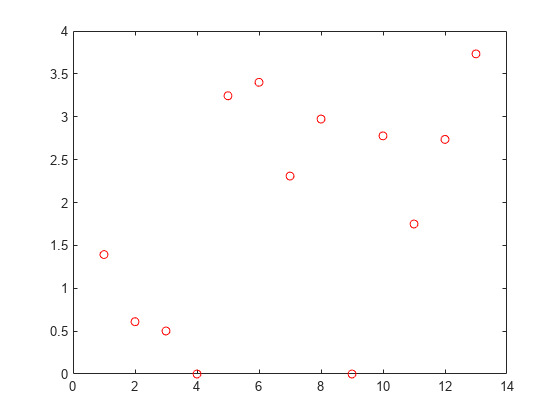The weights of irrelevant features are expected to approach zero. `fsrnca` identifies two features as irrelevant.

Compute the regression loss.

`L = loss(nca,Xtest,ytest,'LossFunction','mad')`
```L = 2.5394 ```

Compute the predicted response values for the test set and plot them versus the actual response.

```ypred = predict(nca,Xtest); figure() plot(ypred,ytest,'bo') xlabel('Predicted response') ylabel('Actual response')```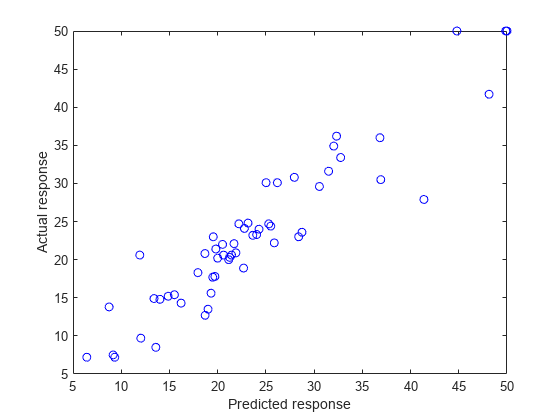A perfect fit versus the actual values forms a 45 degree straight line. In this plot, the predicted and actual response values seem to be scattered around this line. Tuning $\lambda$ (regularization parameter) value usually helps improve the performance.

Tune the regularization parameter using 10-fold cross-validation

Tuning $\lambda$ means finding the $\lambda$ value that will produce the minimum regression loss. Here are the steps for tuning $\lambda$ using 10-fold cross-validation:

1. First partition the data into 10 folds. For each fold, `cvpartition` assigns 1/10th of the data as a training set, and 9/10th of the data as a test set.

```n = length(ytrain); cvp = cvpartition(Xtrain(:,4),'kfold',10); numvalidsets = cvp.NumTestSets;```

Assign the $\lambda$ values for the search. Create an array to store the loss values.

```lambdavals = linspace(0,2,30)*std(ytrain)/n; lossvals = zeros(length(lambdavals),numvalidsets);```

2. Train the neighborhood component analysis (nca) model for each $\lambda$ value using the training set in each fold.

3. Fit a Gaussian process regression (gpr) model using the selected features. Next, compute the regression loss for the corresponding test set in the fold using the gpr model. Record the loss value.

4. Repeat this for each $\lambda$ value and each fold.

``` for i = 1:length(lambdavals) for k = 1:numvalidsets X = Xtrain(cvp.training(k),:); y = ytrain(cvp.training(k),:); Xvalid = Xtrain(cvp.test(k),:); yvalid = ytrain(cvp.test(k),:); nca = fsrnca(X,y,'FitMethod','exact',... 'Lambda',lambdavals(i),... 'Standardize',1,'LossFunction','mad'); % Select features using the feature weights and a relative % threshold. tol = 1e-3; selidx = nca.FeatureWeights > tol*max(1,max(nca.FeatureWeights)); % Fit a non-ARD GPR model using selected features. gpr = fitrgp(X(:,selidx),y,'Standardize',1,... 'KernelFunction','squaredexponential','Verbose',0); lossvals(i,k) = loss(gpr,Xvalid(:,selidx),yvalid); end end```

Compute the average loss obtained from the folds for each $\lambda$ value. Plot the mean loss versus the $\lambda$ values.

```meanloss = mean(lossvals,2); figure; plot(lambdavals,meanloss,'ro-'); xlabel('Lambda'); ylabel('Loss (MSE)'); grid on;```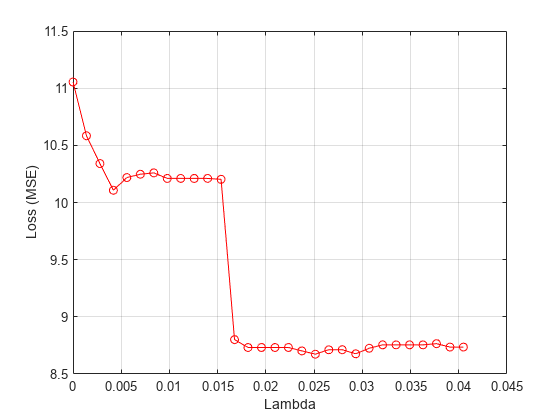Find the $\lambda$ value that produces the minimum loss value.

```[~,idx] = min(meanloss); bestlambda = lambdavals(idx)```
```bestlambda = 0.0251 ```

Perform feature selection for regression using the best $\lambda$ value. Standardize the predictor values.

```nca2 = fsrnca(Xtrain,ytrain,'Standardize',1,'Lambda',bestlambda,... 'LossFunction','mad');```

Plot the feature weights.

```figure() plot(nca.FeatureWeights,'ro')```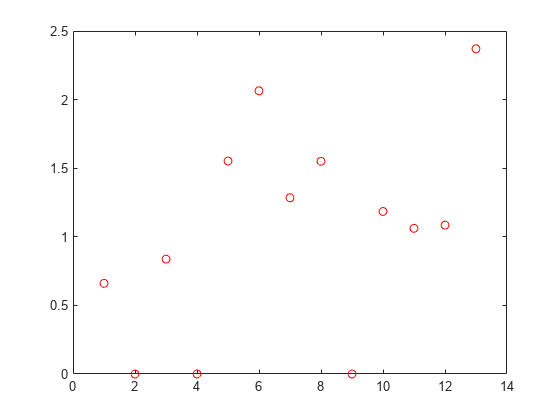Compute the loss using the new nca model on the test data, which is not used to select the features.

`L2 = loss(nca2,Xtest,ytest,'LossFunction','mad')`
```L2 = 2.0560 ```

Tuning the regularization parameter helps identify the relevant features and reduces the loss.

Plot the predicted versus the actual response values in the test set.

```ypred = predict(nca2,Xtest); figure; plot(ypred,ytest,'bo');```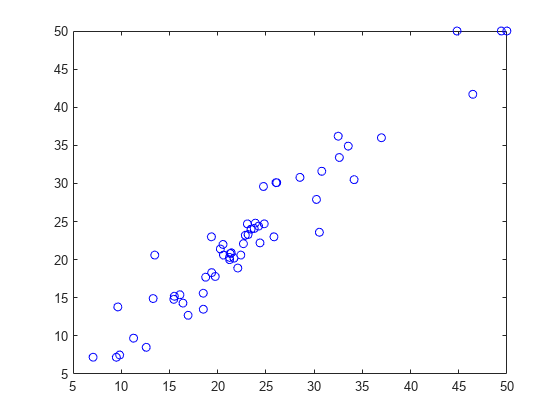The predicted response values seem to be closer to the actual values as well.

References

 Harrison, D. and D.L., Rubinfeld. "Hedonic prices and the demand for clean air." J. Environ. Economics & Management. Vol.5, 1978, pp. 81-102.

 Lichman, M. UCI Machine Learning Repository, Irvine, CA: University of California, School of Information and Computer Science, 2013. https://archive.ics.uci.edu/ml.